StatLect

# Convergence criterion

A convergence criterion is a criterion used to verify the convergence of a sequence.## Statement

The following is a commonly utilized convergence criterion.

Proposition Let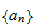be a sequence. Then,where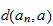is the distance betweenand.

In other words, a sequence is convergent to an elementif and only if the terms of the sequence become closer and closer towhenis increased (closeness is measured by a distance function).

## Example

Consider a sequenceof-dimensional vectors whose generic entry isBecause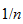and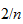converge toasbecomes large, our intuition tells us that the sequenceshould converge to the vectordefined as follows: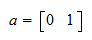How do we verify that this is indeed the case? First of all we need to define a distance functionto measure the distance betweenand a generic term of the sequence. If we use Euclidean distance to measure the distance betweenand, we obtain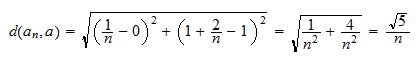But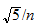converges toby increasing. Therefore, according to the convergence criterion above, the sequenceconverges to.

## More details

Previous entry: Consistent estimator

Next entry: Convolutions